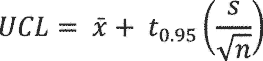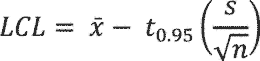# Title 10

Displaying title 10, up to date as of 9/23/2021. Title 10 was last amended 9/22/2021.

# Title 10

###### eCFR Content

The Code of Federal Regulations (CFR) is the official legal print publication containing the codification of the general and permanent rules published in the Federal Register by the departments and agencies of the Federal Government. The Electronic Code of Federal Regulations (eCFR) is a continuously updated online version of the CFR. It is not an official legal edition of the CFR.

§ 429.61 Consumer miscellaneous refrigeration products.

(a) Sampling plan for selection of units for testing.

(1) The requirements of § 429.11 are applicable to miscellaneous refrigeration products; and

(2) For each basic model of miscellaneous refrigeration product, a sample of sufficient size shall be randomly selected and tested to ensure that -

(i) Any represented value of estimated annual operating cost, energy consumption, or other measure of energy consumption of a basic model for which consumers would favor lower values shall be greater than or equal to the higher of:

(A) The mean of the sample, where:And, x is the sample mean; n is the number of samples; and xi is the ith sample; or

(B) The upper 95 percent confidence limit (UCL) of the true mean divided by 1.10, where:And x is the sample mean; s is the sample standard deviation; n is the number of samples; and t0.95 is the t statistic for a 95% one-tailed confidence interval with n-1 degrees of freedom (from appendix A of this subpart).

and

(ii) Any represented value of the energy factor or other measure of energy consumption of a basic model for which consumers would favor higher values shall be less than or equal to the lower of:

(A) The mean of the sample, where:And, x is the sample mean; n is the number of samples; and xi is the ith sample; or

(B) The lower 95 percent confidence limit (LCL) of the true mean divided by 0.90, where:And x is the sample mean; s is the sample standard deviation; n is the number of samples; and t0.95 is the t statistic for a 95% one-tailed confidence interval with n-1 degrees of freedom (from appendix A of this subpart).

(3) The value of total refrigerated volume of a basic model reported in accordance with paragraph (b)(2) of this section shall be the mean of the total refrigerated volumes measured for each tested unit of the basic model or the total refrigerated volume of the basic model as calculated in accordance with § 429.72(d). The value of adjusted total volume of a basic model reported in accordance with paragraph (b)(2) of this section shall be the mean of the adjusted total volumes measured for each tested unit of the basic model or the adjusted total volume of the basic model as calculated in accordance with § 429.72(d).

(b) Certification reports.

(1) The requirements of § 429.12 are applicable to miscellaneous refrigeration products; and

(2) Pursuant to § 429.12(b)(13), a certification report must include the following public product-specific information: The annual energy use in kilowatt hours per year (kWh/yr); the total refrigerated volume in cubic feet (cu ft) and the total adjusted volume in cubic feet (cu ft).

(3) Pursuant to § 429.12(b)(13), a certification report coolers or combination cooler refrigeration products shall include the following additional product-specific information: Whether the basic model has variable defrost control (in which case, manufacturers must also report the values, if any, of CTL and CTM (for an example, see section 5.2.1.3 in appendix A to subpart B of part 430 of this chapter) used in the calculation of energy consumption), whether the basic model has variable anti-sweat heater control (in which case, manufacturers must also report the values of heater Watts at the ten humidity levels 5%, 15%, through 95% used to calculate the variable anti-sweat heater “Correction Factor”), and whether testing has been conducted with modifications to the standard temperature sensor locations specified by the figures referenced in section 5.1 of appendix A to subpart B of part 430 of this chapter.

(c) Rounding requirements for representative values, including certified and rated values.

(1) The represented value of annual energy use must be rounded to the nearest kilowatt hour per year.

(2) The represented value of total refrigerated volume must be rounded to the nearest 0.1 cubic foot.

(3) The represented value of adjusted total volume must be rounded to the nearest 0.1 cubic foot.

(d) Product category determination. Each basic model of miscellaneous refrigeration product must be certified according to the appropriate product category as defined in § 430.2 based on compartment volumes and compartment temperatures.

(1) Compartment volumes used to determine product category shall be the mean of the measured compartment volumes for each tested unit of the basic model according to the provisions in section 5.3 of appendix A to subpart B of part 430 of this chapter, or the compartment volumes of the basic model as calculated in accordance with § 429.72(d); and

(2) Compartment temperatures used to determine product category shall be the mean of the measured compartment temperatures at the coldest setting for each tested unit of the basic model according to the provisions section 5.1 of appendix A to subpart B of part 430 of this chapter. For cooler compartments with temperatures below 39 °F (3.9 °C) but no lower than 37 °F (2.8 °C), the compartment temperatures used to determine product category shall also include the mean of the measured compartment temperatures at the warmest setting for each tested unit of the basic model according to the provisions section 5.1 of appendix A to subpart B of part 430 of this chapter.

[81 FR 46790, July 18, 2016]